NCERT Exemplar: Statistics - 2

# NCERT Exemplar: Statistics - 2 - Mathematics (Maths) Class 10

## Exercise 13.3

Q.1. Find the mean of the distribution :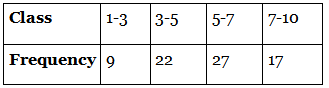Given, the above data represents the class and frequency.
We have to find the mean of the distribution.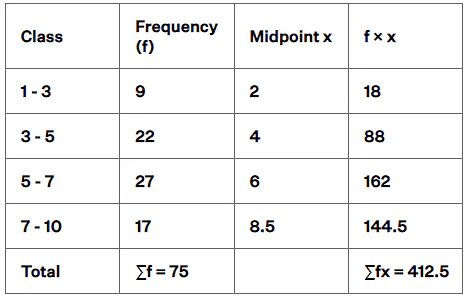By direct method,
Mean = ∑fx / ∑f
From the table,
Mean = 412.5/75
= 5.5
Therefore, the mean is 5.5

Q.2. Calculate the mean of the scores of 20 students in a mathematics test :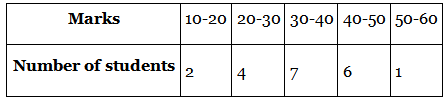Given, the above data represents the marks scored by the number of students in a mathematics test.
We have to determine the mean of the scores of 20 students.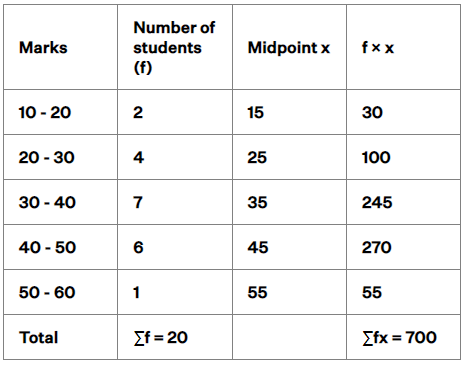By direct method,
Mean = ∑fx / ∑f
From the table,
Mean = 700/20
= 70/2
= 35
Therefore, the mean is 35.

Q.3. Calculate the mean of the following data :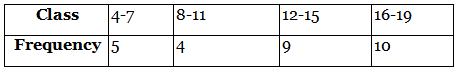Given, the above data gives the class and frequency.
We have to find the mean of the data.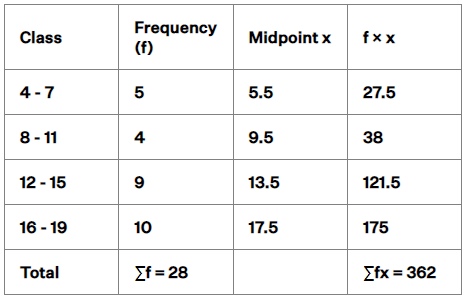By direct method,
Mean = ∑fx / ∑f
From the table,
Mean = 362/28
= 12.928
Therefore, the mean is 12.928

Q. 4. The following table gives the number of pages written by Sarika for completing her own book for 30 days :
Find the mean number of pages written per day.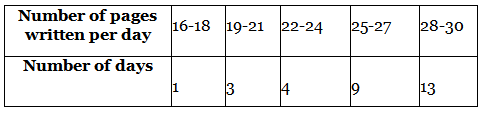Given, the above data gives the number of pages written by Sarika for completing her own book for 30 days.
We have to find the mean number of pages written per day.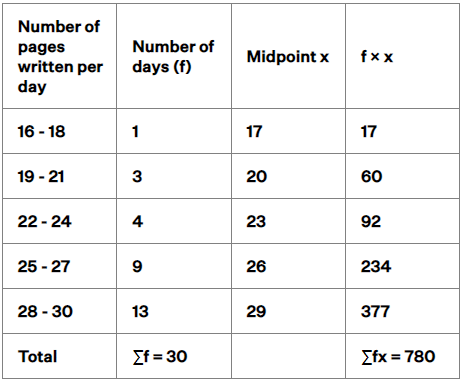By direct method,
Mean = ∑fx / ∑f
From the table,
Mean = 780/30
= 78/3
= 26
Therefore, the mean is 26.

Q.5. The daily income of a sample of 50 employees are tabulated as follows :
Find the mean daily income of employees.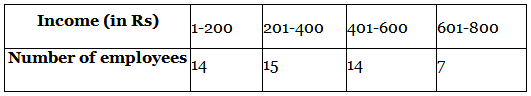Given, the data represents the daily income of a sample of 50 employees.
We have to find the mean daily income of employees.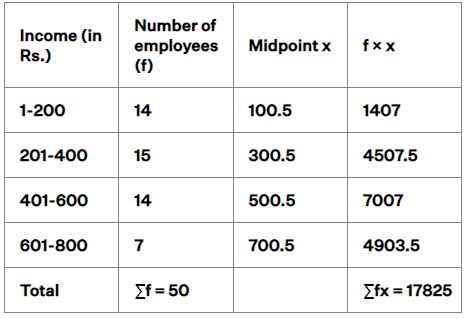By direct method,
Mean = ∑fx / ∑f
From the table,
Mean = 17825/50
= 356.5
Therefore, the mean is 356.5

Q.6. An aircraft has 120 passenger seats. The number of seats occupied during 100 flights is given in the following table :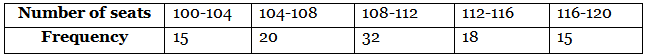Determine the mean number of seats occupied over the flights.

Given, the data represents the seats occupied during 100 flights.
An aircraft has 120 passenger seats.
We have to find the mean number of seats occupied over the flights.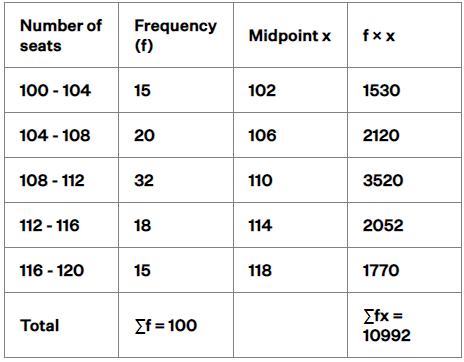By direct method,
Mean = ∑fx / ∑f
From the table,
Mean = 10992/100
= 109.92
Since the seats cannot be in decimal.
Therefore, the mean of seats is 109.92.

Q. 7. The weights (in kg) of 50 wrestlers are recorded in the following table :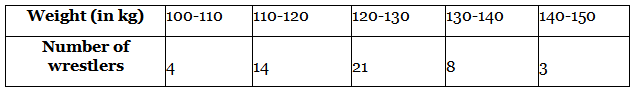Find the mean weight of the wrestlers.

Given, the data represents the weights of 50 wrestlers.
We have to find the mean weight of the wrestlers.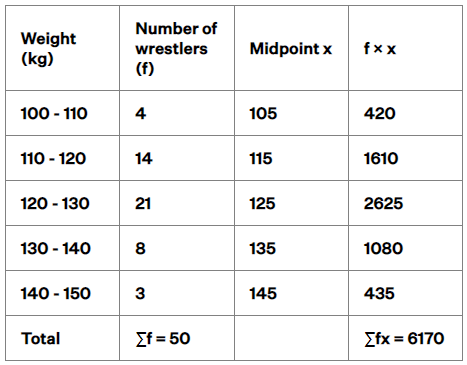By direct method,
Mean = ∑fx / ∑f
From the table,
Mean = 6170/50
= 617/5
= 123.4
Therefore, the mean is 123.4 kg

Q.8. The mileage (km per litre) of 50 cars of the same model was tested by a manufacturer and details are tabulated as given below :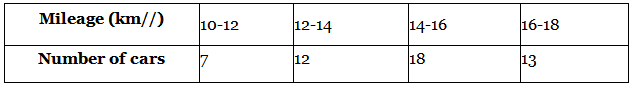Find the mean mileage.The manufacturer claimed that the mileage of the model was 16 km/litre. Do you agree with this claim?

Given, the data represents the mileage of 50 cars of the same model tested by a manufacturer.
We have to find the mean mileage of the cars.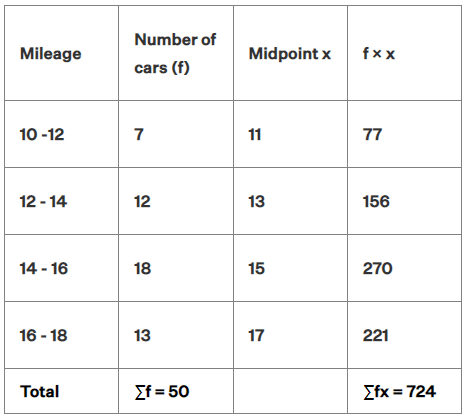By direct method,
Mean = ∑fx / ∑f
From the table,
Mean = 724/50
= 14.48
Therefore, the mean mileage is 14.48 km/litre.
Given, the manufacturer claimed that the mileage of the model was 16 km/litre.
Difference in mileage = 16 - 14.48
= 1.52 km/litre
Therefore, the mean mileage is 1.52 km/litre less than the manufacturers claim.

Q.9. The following is the distribution of weights (in kg) of 40 persons :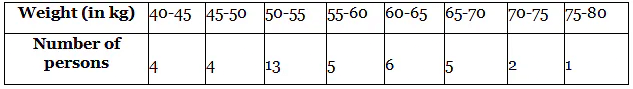Construct a cumulative frequency distribution (of the less than type) table for the data above.

Given, the data represents the weight of 40 persons.
We have to construct a cumulative frequency distribution of the less than type table for the data.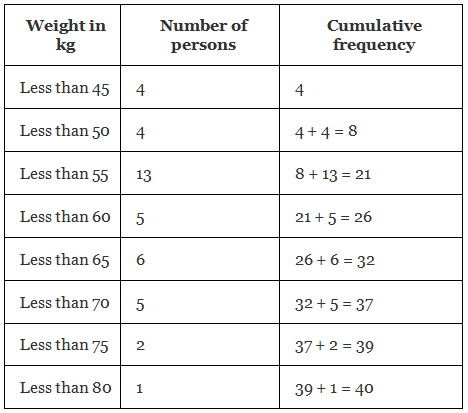Q. 10. The following table shows the cumulative frequency distribution of marks of 800 students in an examination: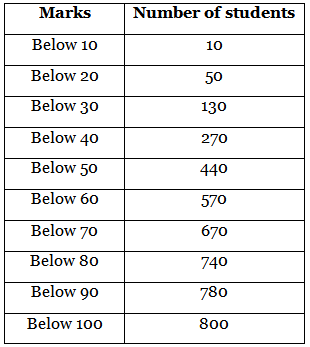Construct a frequency distribution table for the data above.

Given, the data represents the cumulative frequency of marks of 800 students in an examination.
We have to construct a frequency distribution table for the data.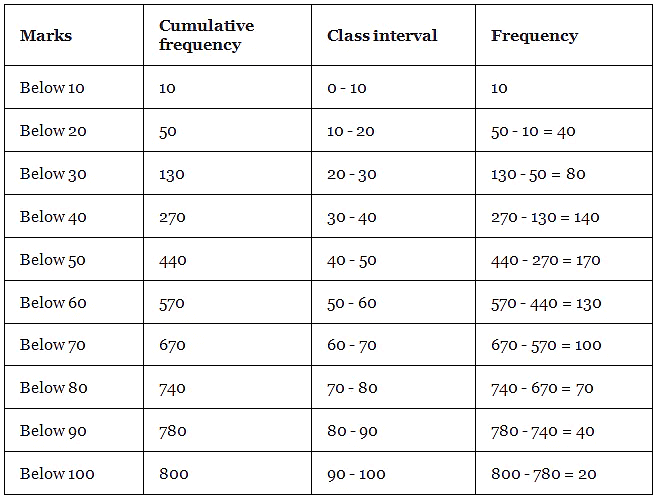Q.11. Form the frequency distribution table from the following data :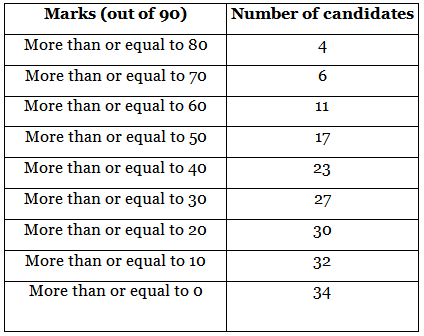Given, the data represents the cumulative frequency of the marks of the candidates.
We have to form the frequency distribution table from the data.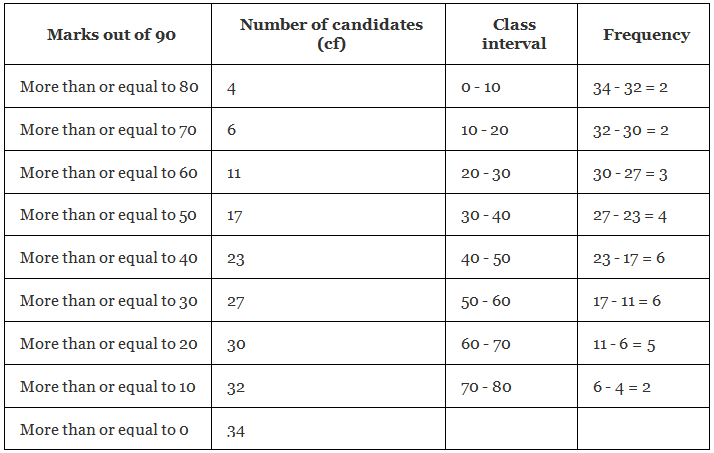Q.12. Find the unknown entries a, b, c, d, e, f  in the following distribution of heights of students in a class :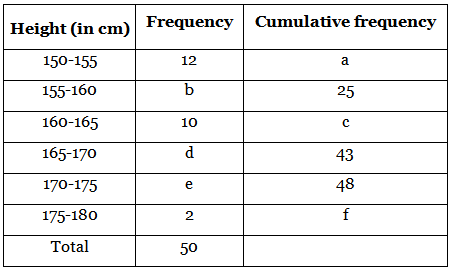Given, the data represents the frequency and cumulative frequency of heights of students in a class.
We have to find the values of the unknown entries a, b, c, d, e, f.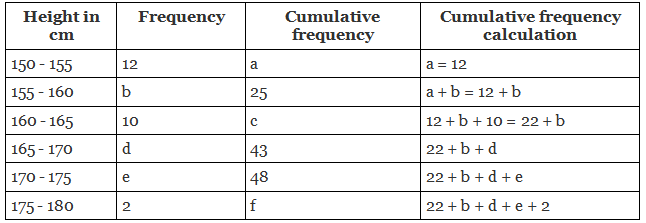From the table,
12 + b = 25

b = 25 - 12
b = 13
22 + b = c
c = 22 + 13
c = 35
22 + b + d = 43
22 + 13 + d = 43
35 + d = 43
d = 43 - 35
d = 8
22 + b + d + e = 48
22 + 13 + 8 + e = 48
35 + 8 + e = 48
43 + e = 48
e = 48 - 43
e = 5
22 + b + d + e + 2 = f
22 + 13 + 8 + 5 + 2 = f
f = 50
Therefore, the values of unknown entries are a = 12, b = 13, c = 35, d = 8, e = 5, f = 50.

Q.13. The following are the ages of 300 patients getting medical treatment in a hospital on a particular day :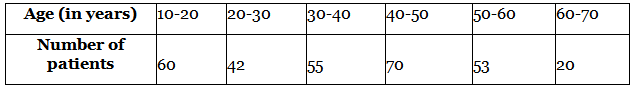Form:(i) Less than type cumulative frequency distribution.
(ii) More than type cumulative frequency distribution.

(i) Given, the data represents the ages of 300 patients getting medical treatment in a hospital on a particular day.
We have to form the cumulative frequency distribution of less than type.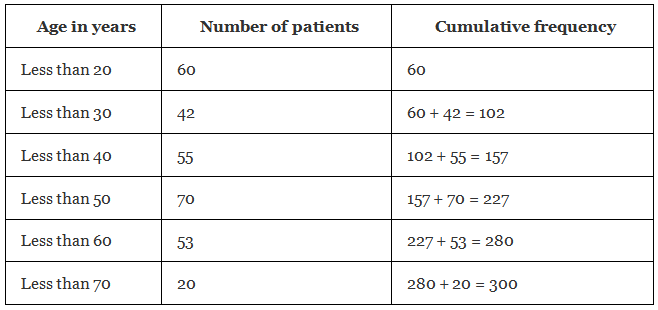(ii) Given, the data represents the ages of 300 patients getting medical treatment in a hospital on a particular day.
We have to form the cumulative frequency distribution of more than type.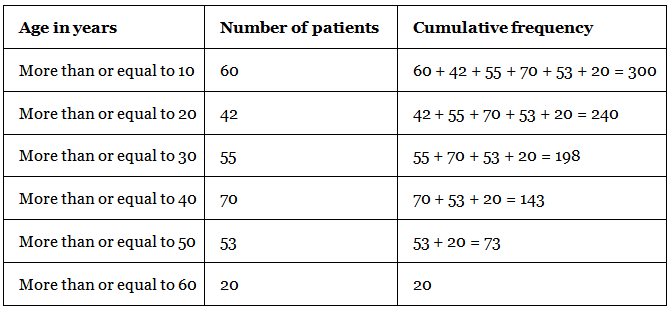Q.14. Given below is a cumulative frequency distribution showing the marks secured by 50 students of a class :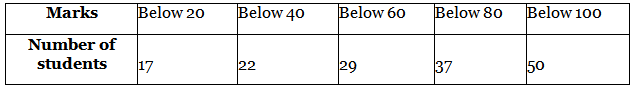Form the frequency distribution table for the data.

Given, the data represents the cumulative frequency distribution of the marks secured by 50 students of a class.
We have to form the frequency distribution table for the data.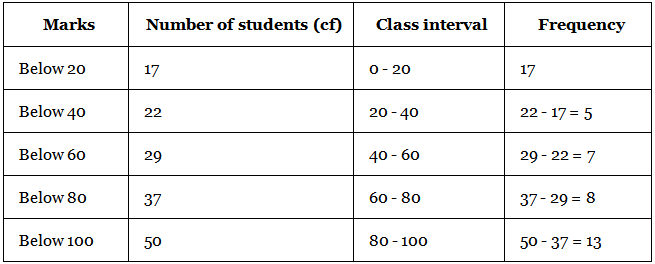Q. 15. Weekly income of 600 families is tabulated below :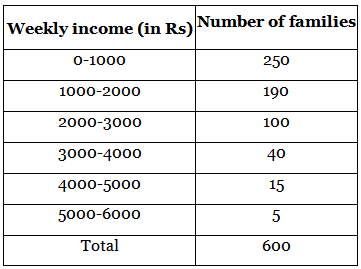Compute the median income.

Given, the weekly income of 600 families.
We have to find the median income.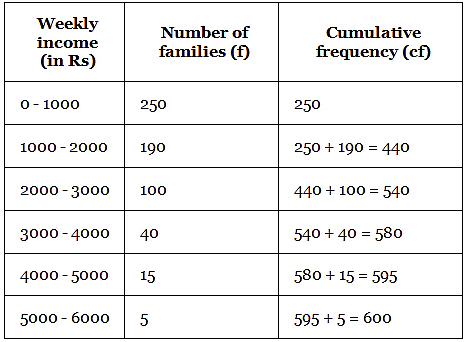Median = l + [(n/2 - cf)/f]h
Where, l is lower limit of the median class
n is the number of observations
h is the class size
cf is the cumulative frequency of the class preceding the median class
f is the frequency of the median class
From the table,
n/2 = 600/2 = 300
The observation lies in the class 1000 - 2000
Lower limit of the class, l = 1000
Class size, h = 1000
Cumulative frequency of the class preceding the median class, cf = 250
Frequency of the median class, f = 190
Median = 1000 + [(300 - 250)/190](1000)
= 1000 + [(50/190)(1000)]
= 1000 + [5(1000)/19]
= 1000 + 263.15
= 1263.15
Therefore, the median is 1263.15

Q.16. The maximum bowling speeds, in km per hour, of 33 players at a cricket coaching centre are given as follows :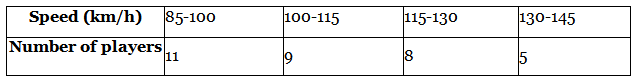Calculate the median bowling speed.

Given, the data represents the maximum bowling speeds of 33 cricket players at a cricket coaching centre.
We have to find the median bowling speed.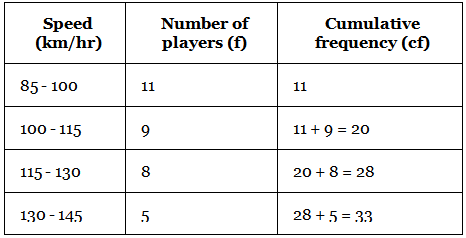Median = l + [(n/2 - cf)/f]h
Where, l is lower limit of the median class
n is the number of observations
h is the class size
cf is the cumulative frequency of the class preceding the median class
f is the frequency of the median class
From the table,
n/2 = 33/2 = 16.5
The observation lies in the class 100 - 115
Lower limit of the class, l = 100
Class size, h = 15
Cumulative frequency of the class preceding the median class, cf = 11
Frequency of the median class, f = 9
Median = 100 + [(16.5 - 11)/9](15)
= 100 + [(5.5/9)(15)]
= 100 + 9.167
= 109.167
Therefore, the median is 109.167.

Q. 17. The monthly income of 100 families are given as below :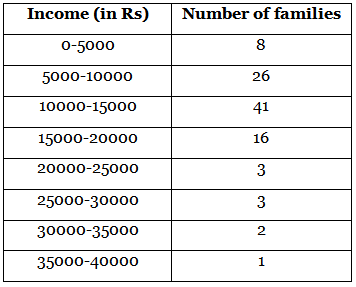Calculate the modal income.

Given, the monthly income of 100 families.
We have to find the modal income.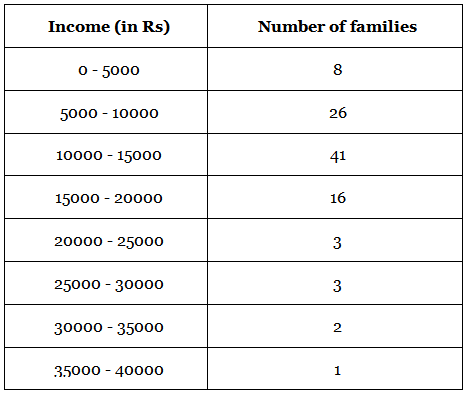Mode = l + [(f₁ - f₀)/(2f₁ - f₀ - f₂)]h
Where, l is the lower limit of the modal class
f₁ is the frequency of the modal class
f₀ is the frequency preceding the modal class
f₂ is the frequency succeeding the modal class
h is the class size
From the table,
Maximum frequency = 41
This frequency lies in the class 10000 - 15000
l = 10000
h = 5000
f₁ = 41
f₀ = 26
f₂ = 16
Now, f₁ - f₀ = 41 - 26 = 15
2f₁ - f₀ - f₂ = 2(41) - 26 - 16
= 82 - 42
= 40
[(f₁ - f₀)/(2f₁ - f₀ - f₂)] = 15/40
= 3/8
Now, mode = 10000 + (3/8)(5000)
= 10000 + (15000/8)
= 10000 + 1875
= 11875
Therefore, the modal income is 11875.

Q. 18. The weight of coffee in 70 packets are shown in the following table :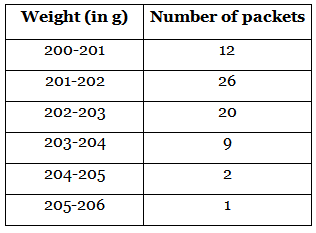Determine the modal weight.

Given, the weight of coffee in 70 packets.
We have to find the modal weight.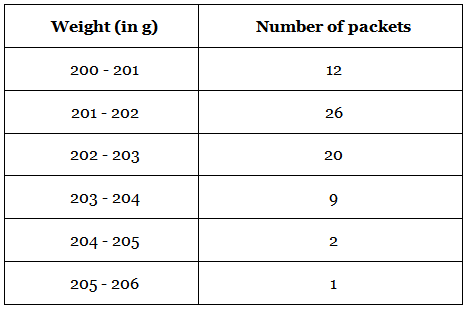Mode = l + [(f₁ - f₀)/(2f₁ - f₀ - f₂)]h
Where, l is the lower limit of the modal class
f₁ is the frequency of the modal class
f₀ is the frequency preceding the modal class
f₂ is the frequency succeeding the modal class
h is the class size
From the table,
Maximum frequency = 26
This frequency lies in the class 201 - 202
l = 201
h = 1
f₁ = 26
f₀ = 12
f₂ = 20
Now, f₁ - f₀ = 26 - 12 = 14
2f₁ - f₀ - f₂ = 2(26) - 12 - 20
= 52 - 32
= 20
[(f₁ - f₀)/(2f₁ - f₀ - f₂)] = 14/20
= 7/10
= 0.7
Now, mode = 201 + 0.7(1)
= 201 + 0.7
= 201.7
Therefore, the mode is 201.7 g

Q.19. Two dice are thrown at the same time. Find the probability of getting
(i) same number on both dice.
(ii) different numbers on both dice.

(i) same number on both dice.
Given, two dice are thrown at the same time.
We have to find the probability of getting the same number on both dice.
When 2 dice are thrown at the same time, the overall possible outcomes are
(1,1) (1,2) (1,3) (1,4) (1,5) (1,6)
(2,1) (2,2) (2,3) (2,4) (2,5) (2,6)
(3,1) (3,2) (3,3) (3,4) (3,5) (3,6)
(4,1) (4,2) (4,3) (4,4) (4,5) (4,6)
(5,1) (5,2) (5,3) (5,4) (5,5) (5,6)
(6,1) (6,2) (6,3) (6,4) (6,5) (6,6)
Total number of possible outcomes = 36
The possibility of getting same number = {(1,1) (2,2) (3,3) (4,4) (5,5) (6,6)}
Number of favourable outcomes = 6
Number of possible outcome = 36
Probability = number of favourable outcomes / number of possible outcomes
Probability of getting the same number = 6/36
= 1/6
Therefore, the probability of getting the same number is 1/6.
(ii) different numbers on both dice.
Given, two dice are thrown at the same time.
We have to find the probability of getting a different number on both dice.
When 2 dice are thrown at the same time, the overall possible outcomes are
(1,1) (1,2) (1,3) (1,4) (1,5) (1,6)
(2,1) (2,2) (2,3) (2,4) (2,5) (2,6)
(3,1) (3,2) (3,3) (3,4) (3,5) (3,6)
(4,1) (4,2) (4,3) (4,4) (4,5) (4,6)
(5,1) (5,2) (5,3) (5,4) (5,5) (5,6)
(6,1) (6,2) (6,3) (6,4) (6,5) (6,6)
Total number of possible outcomes = 36
The possibility of getting different number is
{(1,2) (1,3) (1,4) (1,5) (1,6)
(2,1) (2,3) (2,4) (2,5) (2,6)
(3,1) (3,2) (3,4) (3,5) (3,6)
(4,1) (4,2) (4,3) (4,5) (4,6)
(5,1) (5,2) (5,3) (5,4) (5,6)
(6,1) (6,2) (6,3) (6,4) (6,5)}
Number of favourable outcomes = 30
Number of possible outcome = 36
Probability = number of favourable outcomes / number of possible outcomes
Probability of getting different number = 30/36
= 10/12
= 5/6
Therefore, the probability of getting a different number is 5/6.

Q.20. Two dice are thrown simultaneously. What is the probability that the sum of the numbers appearing on the dice is
(i) 7?

(ii) a prime number?
(iii) 1?

(i) 7
Given, two dice are thrown simultaneously.
We have to find the probability that the sum of the numbers appearing on the dice is 7.
When 2 dice are thrown at the same time, the overall possible outcomes are
(1,1) (1,2) (1,3) (1,4) (1,5) (1,6)
(2,1) (2,2) (2,3) (2,4) (2,5) (2,6)
(3,1) (3,2) (3,3) (3,4) (3,5) (3,6)
(4,1) (4,2) (4,3) (4,4) (4,5) (4,6)
(5,1) (5,2) (5,3) (5,4) (5,5) (5,6)
(6,1) (6,2) (6,3) (6,4) (6,5) (6,6)
Total number of possible outcomes = 36
Favourable outcome = {(1,6) (2,5) (3,4) (4,3) (5,2) (6,1)}
Number of favourable outcomes = 6
Number of possible outcomes = 36
Probability = number of favourable outcomes / number of possible outcomes
Probability of getting a sum of 7 = 6/36
= 1/6
Therefore, the probability of getting the sum of 7 on the dice is 1/6.
(ii) a prime number?
Given, two dice are thrown simultaneously.
We have to find the probability that the sum of the numbers appearing on the dice is a prime number.
When 2 dice are thrown at the same time, the overall possible outcomes are
(1,1) (1,2) (1,3) (1,4) (1,5) (1,6)
(2,1) (2,2) (2,3) (2,4) (2,5) (2,6)
(3,1) (3,2) (3,3) (3,4) (3,5) (3,6)
(4,1) (4,2) (4,3) (4,4) (4,5) (4,6)
(5,1) (5,2) (5,3) (5,4) (5,5) (5,6)
(6,1) (6,2) (6,3) (6,4) (6,5) (6,6)
Total number of possible outcomes = 36
Favourable outcomes = {(1,1) (1,2) (1,4) (1,6) (2,1) (2,3) (2,5) (3,2) (3,4) (4,1) (4,3) (5,2) (5,6) (6,1) (6,5)}
Number of favourable outcomes = 15
Number of possible outcomes = 36
Probability = number of favourable outcomes / number of possible outcomes
Probability = 15/36
= 5/12
Therefore, the probability of getting a sum of a prime number is 5/12.
(iii) 1?
Given, two dice are thrown simultaneously.
We have to find the probability that the sum of the numbers appearing on the dice is 1.
When 2 dice are thrown at the same time, the overall possible outcomes are
(1,1) (1,2) (1,3) (1,4) (1,5) (1,6)
(2,1) (2,2) (2,3) (2,4) (2,5) (2,6)
(3,1) (3,2) (3,3) (3,4) (3,5) (3,6)
(4,1) (4,2) (4,3) (4,4) (4,5) (4,6)
(5,1) (5,2) (5,3) (5,4) (5,5) (5,6)
(6,1) (6,2) (6,3) (6,4) (6,5) (6,6)
Total number of possible outcomes = 36
Number of favourable outcomes = 0
Number of possible outcomes = 36
Probability = number of favourable outcomes / number of possible outcomes
Probability of getting a sum of 1 = 0/36
Therefore, the probability of getting the sum of 1 on the dice is zero.

Q.21. Two dice are thrown together. Find the probability that the product of the numbers on the top of the dice is
(i) 6
(ii) 12
(iii) 7

(i) 6
Given, two dice are thrown together.
We have to find the probability that the product of the numbers on the top of the dice is 6.
When 2 dice are thrown at the same time, the overall possible outcomes are
(1,1) (1,2) (1,3) (1,4) (1,5) (1,6)
(2,1) (2,2) (2,3) (2,4) (2,5) (2,6)
(3,1) (3,2) (3,3) (3,4) (3,5) (3,6)
(4,1) (4,2) (4,3) (4,4) (4,5) (4,6)
(5,1) (5,2) (5,3) (5,4) (5,5) (5,6)
(6,1) (6,2) (6,3) (6,4) (6,5) (6,6)
Total number of possible outcomes = 36
Favourable outcome = {(1,6) (2,3) (3,2) (1,6)}
Number of favourable outcomes = 4
Number of possible outcomes = 36
Probability = number of favourable outcomes / number of possible outcomes
Probability of getting a sum of 7 = 4/36
= 1/9
Therefore, the probability of getting the product of the numbers as 6 on the top of the dice is 1/9.
(ii) 12
Given, two dice are thrown together.
We have to find the probability that the product of the numbers on the top of the dice is 12.
When 2 dice are thrown at the same time, the overall possible outcomes are
(1,1) (1,2) (1,3) (1,4) (1,5) (1,6)
(2,1) (2,2) (2,3) (2,4) (2,5) (2,6)
(3,1) (3,2) (3,3) (3,4) (3,5) (3,6)
(4,1) (4,2) (4,3) (4,4) (4,5) (4,6)
(5,1) (5,2) (5,3) (5,4) (5,5) (5,6)
(6,1) (6,2) (6,3) (6,4) (6,5) (6,6)
Total number of possible outcomes = 36
Favourable outcomes = {(2,6) (3,4) (4,3) (6,2)}
Number of favourable outcomes = 4
Number of possible outcomes = 36
Probability = number of favourable outcomes / number of possible outcomes
Probability = 4/36
= 1/9
Therefore, the probability of getting the product of the numbers as 12 on the top of the dice is 1/9.
(iii) 7
Given, two dice are thrown together.
We have to find the probability that the product of the numbers on the top of the dice is 7.
When 2 dice are thrown at the same time, the overall possible outcomes are
(1,1) (1,2) (1,3) (1,4) (1,5) (1,6)
(2,1) (2,2) (2,3) (2,4) (2,5) (2,6)
(3,1) (3,2) (3,3) (3,4) (3,5) (3,6)
(4,1) (4,2) (4,3) (4,4) (4,5) (4,6)
(5,1) (5,2) (5,3) (5,4) (5,5) (5,6)
(6,1) (6,2) (6,3) (6,4) (6,5) (6,6)
Total number of possible outcomes = 36
Number of favourable outcomes = 0
Number of possible outcomes = 36
Probability = number of favourable outcomes / number of possible outcomes
Probability of getting a sum of 1 = 0/36
Therefore, the probability of getting the product of the numbers on the top of the dice as 7 is zero.

Q.22. Two dice are thrown at the same time and the product of numbers appearing on them is noted. Find the probability that the product is less than 9.

Given, two dice are thrown at the same time.
We have to find the probability that the product of numbers appearing on the dice is less than 9.
When 2 dice are thrown at the same time, the overall possible outcomes are
(1,1) (1,2) (1,3) (1,4) (1,5) (1,6)
(2,1) (2,2) (2,3) (2,4) (2,5) (2,6)
(3,1) (3,2) (3,3) (3,4) (3,5) (3,6)
(4,1) (4,2) (4,3) (4,4) (4,5) (4,6)
(5,1) (5,2) (5,3) (5,4) (5,5) (5,6)
(6,1) (6,2) (6,3) (6,4) (6,5) (6,6)
Total number of possible outcomes = 36
Probability = number of favourable outcomes / number of possible outcomes
The favourable outcomes are
(1,1) (1,2) (1,3) (1,4) (1,5) (1,6)
(2,1) (2,2) (2,3) (2,4)
(3,1) (3,2)
(4,1) (4,2)
(5,1)
(6,1)
Number of favourable outcomes = 16
Number of possible outcomes = 36
Probability = number of favourable outcomes / number of possible outcomes
Probability = 16/36
= 4/9
Therefore, the probability of getting a product less than 9 is 4/9.

Q.23. Two dice are numbered 1, 2, 3, 4, 5, 6 and 1, 1, 2, 2, 3, 3, respectively. They are thrown and the sum of the numbers on them is noted. Find the probability of getting each sum from 2 to 9 separately.

Given, two dice are numbered 1,2,3,4,5,6 and 1,1,2,2,3,3 are thrown and the sum of the numbers on them is noted.
We have to find the probability of getting each sum from 2 to 9 separately.
When the two dice are thrown. The possible outcomes are
(1,1) (1,1) (1,2) (1,2) (1,3) (1,3)
(2,1) (2,1) (2,2) (2,2) (2,3) (2,3)
(3,1) (3,1) (3,2) (3,2) (3,3) (3,3)
(4,1) (4,1) (4,2) (4,2) (4,3) (4,3)
(5,1) (5,1) (5,2) (5,2) (5,3) (5,3)
(6,1) (6,1) (6,2) (6,2) (6,3) (6,3)
Total number of possible outcomes = 36
Probability = number of favourable outcomes / number of possible outcomes
(a) probability of getting a sum of 2
Favourable outcome = {(1,1) (1,1)}
Number of favourbale outcome = 2
Number of possible outcome = 36
Probability = 2/36
= 1/18
Therefore, the probability of getting a sum of 2 is 1/18.
(b) probability of getting a sum of 3
Favourable outcomes = {(1,2) (1,2) (2,1) (2,1)}
Number of favourable outcomes = 4
Number of possible outcomes = 36
Probability = 4/36
= 1/9
Therefore, the probability of getting a sum of 3 is 1/9.
(c) probability of getting a sum of 4
Favourable outcomes = {(1,3) (1,3) (2,2) (2,2), (3, 1), (3, 1)}
Number of favourable outcomes = 6
Number of possible outcomes = 36
Probability = 6/36
= 1/6
Therefore, the probability of getting a sum of 4 is 1/9.
(d) probability of getting a sum of 5
Favourable outcomes = {(2,3) (2,3) (3,2) (3,2) (4,2) (4,2)}
Number of favourable outcomes = 6
Number of possible outcomes = 36
Probability = 6/36
= 1/6
Therefore, the probability of getting a sum of 5 is 1/6.
(e) probability of getting a sum of 6
Favourable outcomes = {(5,1) (5,1) (3,3) (3,3) (4,2) (4,2)}
Number of favourable outcomes = 6
Number of possible outcomes = 36
Probability = 6/36
= 1/6
Therefore, the probability of getting a sum of 6 is 1/6.
(f) probability of getting a sum of 7
Favourable outcomes = {(4,3) (4,3) (5,2) (5,2) (6,1) (6,1)}
Number of favourable outcomes = 6
Number of possible outcomes = 36
Probability = 6/36
= 1/6
Therefore, the probability of getting a sum of 7 is 1/6.
(g) probability of getting a sum of 8
Favourable outcomes = {(5,3) (5,3) (6,2) (6,2)}
Number of favourable outcomes = 4
Number of possible outcomes = 36
Probability = 4/36
= 1/9
Therefore, the probability of getting a sum of 8 is 1/9.
(h) probability of getting a sum of 9
Favourable outcomes = {(6,3)(6,3) }
Number of favourable outcomes = 2
Number of possible outcomes = 36
Probability = 2/36
= 1/18
Therefore, the probability of getting a sum of 9 is 1/18.

Q.24. A coin is tossed two times. Find the probability of getting at most one head.

Given, a coin is tossed two times.
We have to find the probability of getting at most one head.
When a coin is tossed two times.
The possible outcomes are {TT, HH, TH, HT}
Number of possible outcomes = 4
Favourable outcomes = {TT, HT, TH}
Number of favourable outcomes = 3
Probability = number of favourable outcomes / number of possible outcomes
Probability of getting at most one head = 3/4
Therefore, the probability of getting at most one head is 3/4.

Q.25. A coin is tossed 3 times. List the possible outcomes. Find the probability of getting

Given, a coin is tossed 3 times.
We have to find the probability of getting all heads.
When a coin is tossed three times.
The possible outcomes are {TTT, HHH, TTH, THT, THH, HTT, HTH, HHT}
Number of possible outcomes = 8
Favourable outcomes = {HHH)
Number of favourable outcomes = 1
Probability = number of favourable outcomes / number of possible outcomes
Probability of getting all heads = 1/8
Therefore, the probability of getting all heads = 1/8
Given, a coin is tossed 3 times.
We have to find the probability of getting at least 2 heads.
When a coin is tossed three times.
The possible outcomes are {TTT, HHH, TTH, THT, THH, HTT, HTH, HHT}
Number of possible outcomes = 8
Favourable outcomes = {THH, HTH, HHT, HHH}
Number of favourable outcomes = 4
Probability = number of favourable outcomes / number of possible outcomes
Probability of getting all heads = 4/8
Therefore, the probability of getting all heads = 1/2

Q.26. Two dice are thrown at the same time. Determine the probabiity that the difference of the numbers on the two dice is 2.

Given, two dice are thrown at the same time.
We have to determine the probability that the difference of the numbers on the two dice is 2.
When 2 dice are thrown at the same time, the overall possible outcomes are
(1,1) (1,2) (1,3) (1,4) (1,5) (1,6)
(2,1) (2,2) (2,3) (2,4) (2,5) (2,6)
(3,1) (3,2) (3,3) (3,4) (3,5) (3,6)
(4,1) (4,2) (4,3) (4,4) (4,5) (4,6)
(5,1) (5,2) (5,3) (5,4) (5,5) (5,6)
(6,1) (6,2) (6,3) (6,4) (6,5) (6,6)
Total number of possible outcomes = 36
Favourble outcomes to get difference of the numbers on the dice as 2 are
(1,3)
(2,4)
(3,1)(3,5)
(4,2) (4,6)
(5,3)
(6,4)
Number of favourable outcomes = 8
Number of possible outcomes = 36
Probability = number of favourable outcomes / number of possible outcomes
Probability of getting difference of the numbers on the dice as 2 = 8/36
= 4/18
= 2/9
Therefore, the probability of getting the difference of the numbers on the dice as 2 is 2/9.

Q.27. A bag contains 10 red, 5 blue and 7 green balls. A ball is drawn at random. Find the probability of this ball being a

(i) red ball
(ii) green ball
(iii) not a blue ball

(i) red ball
Given, a bag contains 10 red, 5 blue and 7 green balls.
A ball is drawn at random.
We have to find the probability of getting a red ball.
Possible outcomes = 10 red ball + 5 blue ball + 7 green ball
Total number of possible outcomes = 10 + 5 + 7 = 22
The probability of getting a red ball is given by
There are 10 red balls in a bag
Number of favourable outcome = 10
Number of possible outcomes = 22
Probability = number of favourable outcomes / number of possible outcomes
Probability = 10/22
= 5/11
Therefore, the probability of getting a red ball is 5/11.
(ii) green ball
Given, a bag contains 10 red, 5 blue and 7 green balls.
A ball is drawn at random.
We have to find the probability of getting a green ball.
Possible outcomes = 10 red ball + 5 blue ball + 7 green ball
Total number of possible outcomes = 10 + 5 + 7 = 22
The probability of getting a green ball is given by
There are 7 green balls in a bag
Number of favourable outcomes = 7
Number of possible outcomes = 22
Probability = number of favourable outcomes / number of possible outcomes
Probability = 7/22
Therefore, the probability of getting a green ball is 7/22.
(iii) not a blue bal
Given, a bag contains 10 red, 5 blue and 7 green balls.
A ball is drawn at random.
We have to find the probability of getting a red ball, green ball and not a blue ball.
Possible outcomes = 10 red ball + 5 blue ball + 7 green ball
Total number of possible outcomes = 10 + 5 + 7 = 22
The probability of the ball not being a blue ball is given by
There are 10 red balls, 7 green balls and 5 blue balls.
Favourable outcomes = 10 red balls + 7 green balls
Number of favourable outcomes = 17
Number of possible outcomes = 22
Probability = number of favourable outcomes / number of possible outcomes
Probability = 17/22
Therefore, the probability of getting a ball not being a blue ball is 17/22.

Q.28. The king, queen and jack of clubs are removed from a deck of 52 playing cards and then well shuffled. Now one card is drawn at random from the remaining cards. Determine the probability that the card is

(i) a heart
(ii) a king

(i) a heart
Given, the king, queen and jack of clubs are removed from a deck of 52 playing cards and then well shuffled.
We have to determine the probability of drawing a heart.
A deck of 52 playing cards has 13 cards of heart, 13 cards of diamond, 13 cards of spade and 13 cards of club.
King, queen and jack of clubs are removed.
So, remaining cards = 52 - 3 = 49
The probability of drawing a heart is given by
Favourable outcomes = 13 cards of heart
Number of favourable outcomes = 13
Number of possible outcomes = 49
Probability = number of favourable outcomes / number of possible outcomes
Probability = 13/49
Therefore, the probability of drawing a heart is 13/49.
(ii) a king
Given, the king, queen and jack of clubs are removed from a deck of 52 playing cards and then well shuffled.
We have to determine the probability of drawing a king.
A deck of 52 playing cards has 13 cards of heart, 13 cards of diamond, 13 cards of spade and 13 cards of club.
King, queen and jack of clubs are removed.
So, remaining cards = 52 - 3 = 49
The probability of drawing a king is given by
There are 4 kings in a deck.
Given, king of club is removed.
Favourable outcomes = king of heart, king of diamond and king of spade.
Number of favourable outcomes = 3
Number of possible outcomes = 49
Probability = number of favourable outcomes / number of possible outcomes
Probability = 3/49
Therefore, the probability of drawing a king is 3/49

Q. 29. Refer to Q.28. What is the probability that the card is

(i) a club
(ii) 10 of hearts

(i) a club
Given, the king, queen and jack of clubs are removed from a deck of 52 playing cards and then well shuffled.
We have to determine the probability of drawing a club.
A deck of 52 playing cards has 13 cards of heart, 13 cards of diamond, 13 cards of spade and 13 cards of club.
King, queen and jack of clubs are removed.
So, remaining cards = 52 - 3 = 49
We have to find the probability of drawing a club.
There are 13 cards of club.
King, queen and jack are removed.
Remaining cards = 13 - 3 = 10
Favourable outcomes = 10 cards of club
Number of favourable outcomes = 10
Number of possible outcomes = 49
Probability = number of favourable outcomes / number of possible outcomes
Probability = 10/49
Therefore, the probability of drawing a club is 10/49.
(ii) 10 of hearts
Given, the king, queen and jack of clubs are removed from a deck of 52 playing cards and then well shuffled.
We have to determine the probability of drawing a 10 of hearts.
A deck of 52 playing cards has 13 cards of heart, 13 cards of diamond, 13 cards of spade and 13 cards of club.
King, queen and jack of clubs are removed.
So, remaining cards = 52 - 3 = 49
The probability of drawing 10 of hearts is given by
There are 13 cards of heart.
Only one 10 of heart in 13 cards of heart.
Number of favourable outcomes = 1
Number of possible outcomes = 49
Probability = number of favourable outcomes / number of possible outcomes
Probability = 1/49
Therefore, the probability of drawing a 10 of hearts is 1/49

Q.30. All the jacks, queens and kings are removed from a deck of 52 playing cards. The remaining cards are well shuffled and then one card is drawn at random. Giving ace a value 1 similar value for other cards, find the probability that the card has a value

(i) 7
(ii) greater than 7
(iii) less than 7

(i) 7
Given, all the jacks, queens and kings are removed from a deck of 52 playing cards.
The remaining cards are well shuffled and then one card is drawn at random.
Given, ace has a value of 1.
We have to find the probability that the card has a value greater than 7.
A deck of 52 playing cards has 13 cards of heart, 13 cards of diamond, 13 cards of spade and 13 cards of club.
Jacks, queens and kings of heart, spade, diamond and club are removed.
Total number of cards removed = 3(4)
= 12
Remaining cards = 52 - 12
= 40
The probability of drawing a card that has a value 7 is given by
favourable outcomes = card 7 of heart, spade, club and diamond.
Number of favourbale outcomes = 1(4)
= 4
Number of possible outcomes = 40
Probability = number of favourable outcomes / number of possible outcomes
Probability = 4/40
= 1/10
Therefore, the probability of drawing a card that has a value 7 is 1/10.
(ii) greater than 7
Given, all the jacks, queens and kings are removed from a deck of 52 playing cards.
The remaining cards are well shuffled and then one card is drawn at random.
Given, ace has a value of 1.
We have to find the probability that the card has a value greater than 7.
A deck of 52 playing cards has 13 cards of heart, 13 cards of diamond, 13 cards of spade and 13 cards of club.
Jacks, queens and kings of heart, spade, diamond and club are removed.
Total number of cards removed = 3(4)
= 12
Remaining cards = 52 - 12
= 40
The probability of drawing a card greater than 7 is given by
favourable outcomes = 8,9,10 cards of heart, spade, club and diamond.
Number of favourbale outcomes = 3(4)
= 12
Number of possible outcomes = 40
Probability = number of favourable outcomes / number of possible outcomes
Probability = 12/40
= 3/10
Therefore, the probability of drawing a card that has a value greater than 7 is 3/10.
(iii) less than 7
Given, all the jacks, queens and kings are removed from a deck of 52 playing cards.
The remaining cards are well shuffled and then one card is drawn at random.
Given, ace has a value of 1.
We have to find the probability that the card has a value less than 7.
A deck of 52 playing cards has 13 cards of heart, 13 cards of diamond, 13 cards of spade and 13 cards of club.
Jacks, queens and kings of heart, spade, diamond and club are removed.
Total number of cards removed = 3(4)
= 12
Remaining cards = 52 - 12
= 40
The probability of drawing a card less than 7 is given by
favourable outcomes = 1,2,3,4,5,6 cards of heart, spade, club and diamond.
Number of favourbale outcomes = 6(4)
= 24
Number of possible outcomes = 40
Probability = number of favourable outcomes / number of possible outcomes
Probability = 24/40
= 6/10
= 3/5
Therefore, the probability of drawing a card that has a value less than 7 is 3/5.

Q.31. An integer is chosen between 0 and 100. What is the probability that it is

(i) divisible by 7?
(ii) not divisible by 7?

(i) divisible by 7?
Given, an integer is chosen between 0 and 100.
We have to find the probability that an integer chosen is divisible by 7.
Integers between 0 and 100 = 1, 2, 3, 4,........and 99
Total number of integers between 0 and 100 = 99.
The probability that an integer chosen is divisible by 7 is given by
Favourbale outcomes = 7, 14, 21, 28, 35, 42, 49, 56, 63, 70, 77, 84, 91, 98
Number of favourable outcomes = 14
Number of possible outcomes = 99
Probability = number of favourable outcomes / number of possible outcomes.
Probability = 14/99
Therefore, the probability of choosing an integer that is divisible by 7 is 14/99
(ii) not divisible by 7?
Given, an integer is chosen between 0 and 100.
We have to find the probability that an integer chosen is divisible by 7.
Integers between 0 and 100 = 1, 2, 3, 4,........and 99
Total number of integers between 0 and 100 = 99.
The probability that an integer chosen is not divisible by 7 is given by
Integers divisible by 7 between 0 and 100 = 7, 14, 21, 28, 35, 42, 49, 56, 63, 70, 77, 84, 91, 98
Number of integers divisible by 7 = 14
Favourable outcomes = Integers not divisible by 7
Integers not divisible by 7 = total number of integers between 0 and 100 - number of integers divisible divisible by 7 between 0 and 100.
= 99 - 14
= 85
Number of favourable outcomes = 85
Number of possible outcomes = 99
Probability = number of favourable outcomes / number of possible outcomes.
Probability = 85/99
Therefore, the probability of choosing an integer that is not divisible by 7 is 85/99.

Q.32. Cards with numbers 2 to 101 are placed in a box. A card is selected at random.
Find the probability that the card has
(i) an even number
(ii) a square number

(i) an even number
Given, cards with numbers 2 to 101 are placed in a box.
A card is selected at random.
We have to find the probability that the card has an even number.
Total number cards = 100
Using arithmetic progression to find the number of cards with even numbers.
The last term is given by l = a + (n - 1)d
Where, l is the last term
a is the first term
n is the number of terms
d is the common difference
Given, cards with numbers 2 to 101. We need a total number of even numbers in the given cards.
a = 2
l = 100
d = 2
So, 100 = 2 + (n - 1)2
100 - 2 = 2n - 2
100 - 2 + 2 = 2n
100 = 2n
n = 100/2
n = 50
Number of favourable outcomes = 50
Number of possible outcomes = 100
Probability = number of favoruable outcomes / number of possible outcomes
Probability = 50/100
= 5/10
= 1/2
Therefore, the probability of selecting a card that has an even number is 1/2.
(ii) a square number
Given, cards with numbers 2 to 101 are placed in a box.
A card is selected at random.
We have to find the probability that the card has a square number.
The probability of selecting a card that has a square number is given by
Cards with square numbers between 2 to 101 = (2)², (3)², (4)², (5)², (6)², (7)², (8)², (9)², (10)²
Favourable outcomes = 4, 9, 16, 25, 36, 49, 64, 81, 100
Number of favourable outcomes = 9
Number of possible outcomes = 100
Probability = number of favoruable outcomes / number of possible outcomes
Probability = 9/100
Therefore, the probability of selecting a card that has a square number is 9/100.

Q.33. A letter of English alphabets is chosen at random. Determine the probability that the letter is a consonant.

Given, a letter of English alphabets is chosen at random.
We have to determine the probability that the letter is a consonant.
There are 26 English alphabets which consist of 5 vowels and 21 consonants.
The probability of selecting a letter that is a consonant is given by
Favourable outcomes = b, c, d, f, g, h , j, k , l, m, n, p, q, r, s, t, v, w, x, y, z
Number of favourable outcomes = 21
Number of possible outcomes = 26
Probability = number of favourable outcomes / number of possible outcomes
Probability = 21/26
Therefore, the probability of choosing an alphabet that is a consonant is 21/26.

Q.34. There are 1000 sealed envelopes in a box, 10 of them contain a cash prize of Rs 100 each, 100 of them contain a cash prize of Rs 50 each and 200 of them contain a cash prize of Rs 10 each and rest do not contain any cash prize. If they are well shuffled and an envelope is picked up out, what is the probability that it contains no cash prize?

Given, a box contains 1000 sealed envelopes.
10 of them contain a cash prize of Rs. 100 each.
100 of them contain a cash prize of Rs. 50 each.
200 of them contain a cash prize of Rs. 10 each.
Rest do not contain any cash prize.
The box is well shuffled and an envelope is picked out.
We have to find the probability that the envelope contains no cash prize.
The probability of picking out an envelope that it contains no cash prize is given by
Favourable outcomes = Envelopes that do not contain cash prize
Envelopes that do not contain cash prize = total envelopes - envelopes that contain Rs. 100 - envelopes that contain Rs. 50 - envelopes that contain Rs. 10
= 1000 - 10 - 100 - 200
= 1000 - 310
= 690
Number of favourable outcomes = 690
Number of possible outcomes = 1000
Probability = number of favourable outcomes / number of possible outcomes
Probability = 690/100
= 69/100
Therefore, the probability of selecting an envelope that contains no cash prize is 69/100.

Q.35. Box A contains 25 slips of which 19 are marked Re 1 and other are marked Rs 5 each. Box B contains 50 slips of which 45 are marked Re 1 each and others are marked Rs 13 each. Slips of both boxes are poured into a third box and resuffled.  A slip is drawn at random. What is the probability that it is marked other than Re 1?

Given, box A contains 25 slips of which 19 are marked Re. 1 and other are marked Rs. 5 each.
Box B contains 50 slips of which 45 are marked Re. 1 each and others are marked Rs. 13 each.
Slips of both boxes are poured into a third box and reshuffled.
A slip is drawn at random.
We have to find the probability of selecting a slip that is marked other than Re. 1.
Considering box A,
Total number of slips = 25
19 slips are marked Re. 1
Slips marked Rs. 5 = 25 - 19 = 6
Considering box B,
Total number of slips = 50
45 slips are marked with Re. 1
Slips marked Rs. 13 = 50 - 45 = 5
Considering third box,
Slips marked Re. 1 = 19 + 45 = 64
Slips marked Rs. 5 = 6
Slips marked Rs. 13 = 5
Total number of slips = 25 + 50 = 75
Favourable outcomes = slips marked other than Re.1
Slips marked other than Re. 1 = slips marked Rs. 5 and slips marked Rs. 13
Number of favourable outcomes = 6 + 5 = 11
Number of possible outcomes = 75
Probability = number of favourable outcomes / number of possible outcomes
Probability = 11/75
Therefore, the probability of selecting a slip that is marked other than Re.1 is 11/75.

Q.36. A carton of 24 bulbs contain 6 defective bulbs. One bulbs is drawn at random.

What is the probability that the bulb is not defective? If the bulb selected is defective and it is not replaced and a second bulb is selected at random from the rest, what is the probability that the second bulb is defective?

Given, a carton of 24 bulbs contain 6 defective bulbs.
One bulb is drawn at random.
We have to find the probability that the bulb is not defective.
Favourable outcomes = bulbs which are not defective
Bulbs which are not defective = 24 - 6
= 18
Number of favourable outcomes = 18
Number of possible outcomes = 24
Probability = number of favourable outcomes / number of possible outcomes
Probability = 18/24
= 3/4
Therefore, the probability of selecting a bulb that is not defective is 3/4.
The bulb selected is defective and it is not replaced.
A second bulb is selected at random from the rest.
We have to find the probability of selecting a second bulb that is defective.
Now, total number of bulbs = 23
Favourable outcomes = defective bulb
Number of defective bulbs = 6 - 1 = 5
Number of favourable outcomes = 5
Number of possible outcomes = 23
Probability = number of favourable outcomes / number of possible outcomes
Probability = 5/23
Therefore, the probability of selecting a second bulb that is defective is 5/23.

Q.37. A child’s game has 8 triangles of which 3 are blue and rest are red, and 10 squares of which 6 are blue and rest are red. One piece is lost at random. Find the probability that it is a

(i) triangle
(ii) square
(iii) square of blue colour
(iv) triangle of red colour

(i) triangle
Given, a child’s game has 8 triangles of which 3 are blue and the rest are red.
10 squares of which are blue and the rest are red.
One piece is lost at random.
We have to find the probability that it is a triangle.
Considering 8 triangles,
Number of blue triangles = 3
Number of red triangles = 8 - 3 = 5
Considering 10 squares,
Number of blue squares = 6
Number of red squares = 10 - 6 = 4
Total number of game = 8 + 10 = 18
The probability that the lost piece is a triangle is given by
Favourable outcome = triangle
Number of favourable outcomes = 8
Number of possible outcomes = 18
Probability = number of favourable outcomes / number of possible outcomes
Probability = 8/18
= 4/9
Therefore, the probability that the lost piece is a triangle is 4/9.
(ii) square
Given, a child’s game has 8 triangles of which 3 are blue and the rest are red.
10 squares of which are blue and rest are red.
One piece is lost at random.
We have to find the probability that it is a square.
Considering 8 triangles,
Number of blue triangles = 3
Number of red triangles = 8 - 3 = 5
Considering 10 squares,
Number of blue squares = 6
Number of red squares = 10 - 6 = 4
Total number of game = 8 + 10 = 18
The probability that the lost piece is a square is given by
Favourable outcome = square
Number of favourable outcomes = 10
Number of possible outcomes = 18
Probability = number of favourable outcomes / number of possible outcomes
Probability = 10/18
= 5/9
Therefore, the probability that the lost piece is a square is 5/9.
(iii) square of blue colour
Given, a child’s game has 8 triangles of which 3 are blue and the rest are red.
10 squares of which are blue and the rest are red.
One piece is lost at random.
We have to find the probability that it is a square of blue colour.
Considering 8 triangles,
Number of blue triangles = 3
Number of red triangles = 8 - 3 = 5
Considering 10 squares,
Number of blue squares = 6
Number of red squares = 10 - 6 = 4
Total number of game = 8 + 10 = 18
The probability that the lost piece is a square of blue colour is given by
Favourable outcome = square of blue colour
Number of favourable outcomes = 6
Number of possible outcomes = 1
Probability = number of favourable outcomes / number of possible outcomes
Probability = 6/18
= 1/3
Therefore, the probability that the lost piece is a square of blue colour is 1/3.
(iv) triangle of red colour
Given, a child’s game has 8 triangles of which 3 are blue and rest are red.
10 squares of which are blue and rest are red.
One piece is lost at random.
We have to find the probability that it is a triangle of red colour.
Considering 8 triangles,
Number of blue triangles = 3
Number of red triangles = 8 - 3 = 5
Considering 10 squares,
Number of blue squares = 6
Number of red squares = 10 - 6 = 4
Total number of game = 8 + 10 = 18
The probability that the lost piece is a triangle of red colour is given by
Favourable outcome = triangle of red colour
Number of favourable outcomes = 5
Number of possible outcomes = 18
Probability = number of favourable outcomes / number of possible outcomes
Probability = 5/18
Therefore, the probability that the lost piece is a triangle of red colour is 5/18.

Q.38. In a game, the entry fee is Rs 5. The game consists of a tossing a coin 3 times. If one or two heads show, Sweta gets her entry fee back. If she throws 3 heads, she receives double the entry fees. Otherwise she will lose. For tossing a coin three times, find the probability that she
(i) loses the entry fee.
(ii) gets double entry fee.
(iii) just gets her entry fee.

(i) loses the entry fee.
Given, the entry fee in a game is Rs. 5
The game consists of tossing a coin 3 times.
If one or two heads show up, Sweta gets her entry fee back.
Otherwise she will lose.
We have to find the probability that she loses the entry fee.
When a coin is tossed three times.
The possible outcomes are {TTT, HHH, TTH, THT, THH, HTT, HTH, HHT}
Number of possible outcomes = 8
Favourable outcomes = Sweta loses when she throws 3 tails = TTT
Number of favoruable outcomes = 1
Probability = number of favourable outcomes / number of possible outcomes
Probability = 1/8
Therefore, the probability that Sweta loses her entry fee is 1/8.
(ii) gets double entry fee.
Given, the entry fee in a game is Rs. 5
The game consists of tossing a coin 3 times.
If one or two heads show up, Sweta gets her entry fee back.
Otherwise she will lose.
We have to find the probability that she gets double entry fee.
When a coin is tossed three times.
The possible outcomes are {TTT, HHH, TTH, THT, THH, HTT, HTH, HHT}
Number of possible outcomes = 8
Favourable outcomes = when she throws 3 heads = HHH
Number of favoruable outcomes = 1
Probability = number of favourable outcomes / number of possible outcomes
Probability = 1/8
Therefore, the probability that Sweta gets double entry fee is 1/8.
(iii) just gets her entry fee.
Given, the entry fee in a game is Rs. 5
The game consists of tossing a coin 3 times.
If one or two heads show up, Sweta gets her entry fee back.
Otherwise she will lose.
We have to find the probability that she just gets her entry fee.
When a coin is tossed three times.
The possible outcomes are {TTT, HHH, TTH, THT, THH, HTT, HTH, HHT}
Number of possible outcomes = 8
Favourable outcomes = when she throws one or heads
= TTH, THT, THH, HTT, HTH, HHT
Number of favoruable outcomes = 6
Probability = number of favourable outcomes / number of possible outcomes
Probability = 6/8
= 3/4
Therefore, the probability that Sweta just gets her entry fee is 3/4.

Q.39. A die has its six faces marked 0, 1, 1, 1, 6, 6. Two such dice are thrown together and the total score is recorded.

(i) How many different scores are possible?
(ii) What is the probability of getting a total of 7?

(i) How many different scores are possible?
Given, a die has its six faces marked 0, 1, 1, 1, 6, 6.
Two such dice are thrown together and the total score is recorded.
We have to find how many different scores are possible.
When two dice are thrown, the possible outcomes are
(0,0) (0,1) (0,1) (0,1) (0,6) (0,6)
(1,0) (1,1) (1,1) (1,1) (1,6) (1,6)
(1,0) (1,1) (1,1) (1,1) (1,6) (1,6)
(1,0) (1,1) (1,1) (1,1) (1,6) (1,6)
(6,0) (6,1) (6,1) (6,1) (6,6) (6,6)
(6,0) (6,1) (6,1) (6,1) (6,6) (6,6)
Number of possible outcomes = 36
Scores which are possible = 0, 1, 2, 6, 7 and 12.
Number of scores which are possible = 6
Therefore, the number of scores is 6
(ii) What is the probability of getting a total of 7?
Given, a die has its six faces marked 0, 1, 1, 1, 6, 6. 0, 1, 1, 1, 6, 6.
Two such dice are thrown together and the total score is recorded.
We have to find the probability of getting a total of 7.
When two dice are thrown, the possible outcomes are
(0,0) (0,1) (0,1) (0,1) (0,6) (0,6)
(1,0) (1,1) (1,1) (1,1) (1,6) (1,6)
(1,0) (1,1) (1,1) (1,1) (1,6) (1,6)
(1,0) (1,1) (1,1) (1,1) (1,6) (1,6)
(6,0) (6,1) (6,1) (6,1) (6,6) (6,6
(6,0) (6,1) (6,1) (6,1) (6,6) (6,6)
Number of possible outcomes = 36
Favourable outcome = total of 7
= (1,6) (1,6) (1,6) (1,6) (1,6) (1,6) (6,1) (6,1) (6,1) (6,1) (6,1) (6,1)
Number of favourable outcomes = 12
Probability = number of favourable outcomes / number of possible outcomes
Probability = 12/3
= 1/3
Therefore, the probability of getting a total of 7 is 1/3.

Q.40. A lot consists of 48 mobile phones of which 42 are good, 3 have only minor defects and 3 have major defects. Varnika will buy a phone if it is good but the trader will only buy a mobile if it has no major defect. One phone is selected at random from the lot. What is the probability that it is
(i) acceptable to Varnika?

(i) acceptable to Varnika?
Given, a lot consists of 48 mobile phones of which 42 are good, 3 have only minor defects and 3 have major defects.
Varnika will buy a phone if it is good but the trader will only buy a mobile if it has no major defect.
One phone is selected at random from the lot.
We have to find the probability of selecting a phone that is acceptable to Varnika.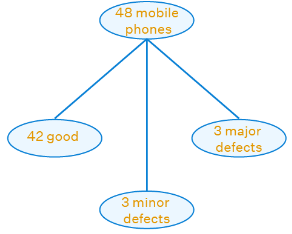Favourable outcome = good phonesVarnika will buy only good phones.
Number of favourable outcome = 42
Number of possible outcomes = 48
Probability = number of favourable outcomes / number of possible outcomes
Probability = 42/48
= 7/8
Therefore, the probability of selecting a good phone is 7/8.
Given, a lot consists of 48 mobile phones of which 42 are good, 3 have only minor defects and 3 have major defects.
Varnika will buy a phone if it is good but the trader will only buy a mobile if it has no major defect.
One phone is selected at random from the lot.
We have to find the probability of selecting a phone that is acceptable to the traderFavourable outcome = phone acceptable to the trader.
Phone acceptable to the trader = phone which is good + phone which has no major defects
Number of favourable outcome = 42 + 3 = 45
Number of possible outcomes = 48
Probability = number of favourable outcomes / number of possible outcomes
Probability = 45/48
= 15/16
Therefore, the probability of selecting a phone acceptable to the trader is 15/16.

Q.41. A bag contains 24 balls of which x are red, 2x are white and 3x are blue. A ball is selected at random. What is the probability that it is

(i) not red?
(ii) white?

(i) not red?
Given, a bag contains 24 balls of which x are red, 2x are white and 3x are blue.
A ball is selected at random.
We have to find the probability of selecting a ball that is not red.
Given, x + 2x + 3x = 24
6x = 24
x = 24/6
x = 4
Number of red balls = x
= 4
Number of white balls = 2x
= 2(4)
= 8
Number of blue balls = 3x
= 3(4)
= 12
The probability of selecting a ball that is not red is given by
Favourbale outcomes = balls other than red
= white balls + blue balls
Number of favourable outcomes = 8 + 12 = 20
Number of possible outcomes = 24
Probability = number of favourable outcomes / number of possible outcomes
Probability = 20/24
= 10/12
= 5/6
Therefore, the probability of selecting a ball that is not red is 5/6.
(ii) white?
Given, a bag contains 24 balls of which x are red, 2x are white and 3x are blue.
A ball is selected at random.
We have to find the probability of selecting a ball that is white.
Given, x + 2x + 3x = 24
6x = 24
x = 24/6
x = 4
Number of red balls = x
= 4
Number of white balls = 2x
= 2(4)
= 8
Number of blue balls = 3x
= 3(4)
= 12
The probability of selecting a ball that is white is given by
Favourbale outcomes = white balls
Number of favourable outcomes = 8
Number of possible outcomes = 24
Probability = number of favourable outcomes / number of possible outcomes
Probability = 8/24
= 1/3
Therefore, the probability of selecting a ball that is white is 1/3.

Q.42. At a fete, cards bearing numbers 1 to 1000, one number on one card, are put in a box. Each player selects one card at random and that card is not replaced. If the selected card has a perfect square greater than 500, the player wins a prize. What is the probability that
(i) the first player wins a prize?
(ii) the second player wins a prize, if the first has won?

(i) the first player wins a prize?
Given, at a fete, cards bearing numbers 1 to 1000, one number on one card, are put in a box.
Each player selects one card at random and that card is not replaced.
If the selected square has a perfect square greater than 500, the player wins a prize.
We have to find the probability that the first player wins a prize.
Given, cards with numbers 1 to 1000.
Total number of cards = 1000
The probability that the first player wins a prize is given by
Favourable outcome = player selects a perfect square greater than 500.
Perfect square greater than 500 = {(23)², (24)², (25)², (26)², (27)², (28)², (29)², (30)², (31)²}
= {529, 576, 625, 676, 729, 784, 841, 900, 961}
Number of favourable outcomes = 9
Number of possible outcomes = 1000
Probability = number of favourable outcomes / number of possible outcomes
Probability = 9/1000
Therefore, the probability that the first player wins a prize is 9/1000.
(ii) the second player wins a prize, if the first has won?
Given, at a fete, cards bearing numbers 1 to 1000, one number on one card, are put in a box.
Each player selects one card at random and that card is not replaced.
If the selected square has a perfect square greater than 500, the player wins a prize.
We have to find the probability that the second player wins a prize.
Given, cards with numbers 1 to 1000.
Total number of cards = 1000
The first player wins a prize by choosing a perfect square number.
The card is not replaced.
Remaining cards = 1000 - 1 = 999
The probability that the second player wins a prize is given by
Favourable outcome = player selects a perfect square greater than 500 except the card chosen by first player.
Perfect square greater than 500 = {(23)², (24)², (25)², (26)², (27)², (28)², (29)², (30)², (31)²}
= {529, 576, 625, 676, 729, 784, 841, 900, 961}
Number of favourable outcomes = 9 - 1 = 8
Number of possible outcomes = 999
Probability = number of favourable outcomes / number of possible outcomes
Probability = 8/999
Therefore, the probability that the second player wins a prize is 8/999

The document NCERT Exemplar: Statistics - 2 | Mathematics (Maths) Class 10 is a part of the Class 10 Course Mathematics (Maths) Class 10.
All you need of Class 10 at this link: Class 10

## Mathematics (Maths) Class 10

115 videos|478 docs|129 tests

## Mathematics (Maths) Class 10

115 videos|478 docs|129 testsExplore Courses for Class 10 examSignup to see your scores go up within 7 days! Learn & Practice with 1000+ FREE Notes, Videos & Tests.
10M+ students study on EduRev
Track your progress, build streaks, highlight & save important lessons and more!
Related Searches

,

,

,

,

,

,

,

,

,

,

,

,

,

,

,

,

,

,

,

,

,

;